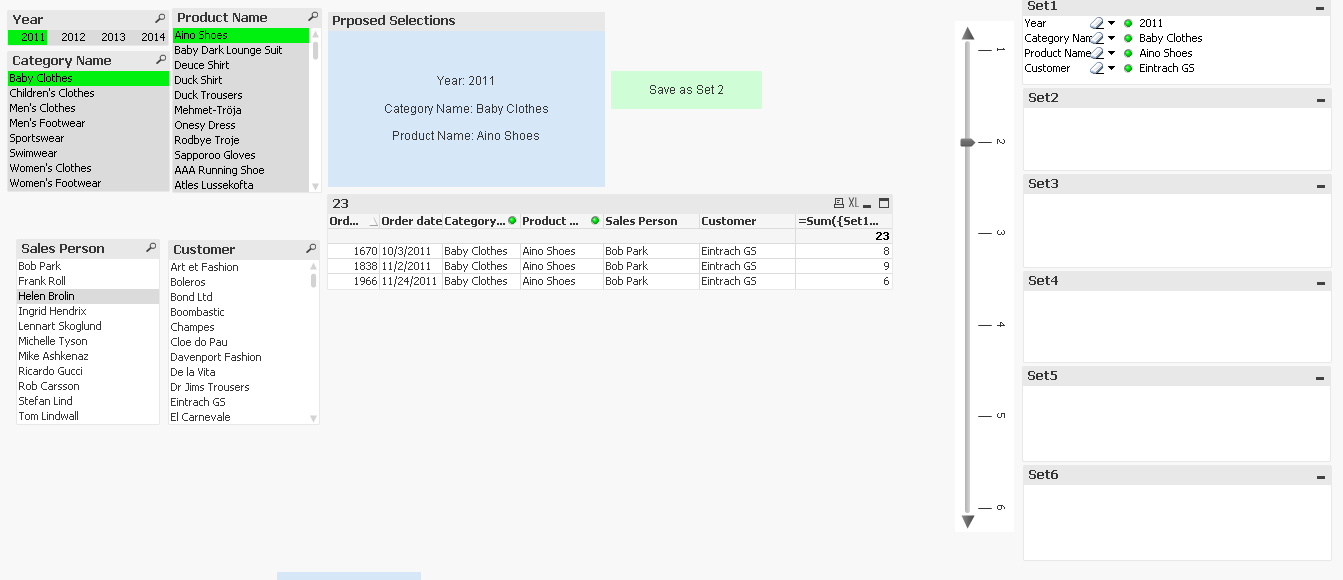# New to QlikView

Discussion board where members can get started with QlikView.

Announcements
QlikWorld, June 24-25, 2020. Free virtual event for DI and DA gurus. Register Now
cancel
Showing results for
Did you mean:
HighlightedCreator II

## How to show the sum of a column for different alternate states in one Expression

Hello Community,

Here, I am having a Query:

I have 6 alternate states named as set1, set2, set3, set4, set5, set6 (These 6 are Current Selection boxes i.e i used state name function) and i have 4 list boxes named as year, Category, Product name, Customer (Now these 4 are with another alternate state named as 'proposed').

Now i have created one straight table with these columns year, Category, Product name, Customer, Sum(Quantity).

But, i want to show the values when we pass our selections to that sets those values only i want to see.

for that i used sum({Set1 + Set2 + Set3 + Set4 + Set5 + Set6} Quantity). Here, also i am getting problem.. when i pass the selections to all sets that time only it is giving correct result.

My requirement is if i pass my selections to only set1 then also i wnat correct result / if pass the selections to 2 or 3 sets then that time also i want correct result

For that How can i write my expression can you give me the suggestion apart from i used these below expressions also:

1.

=sum({<[Sales Person]=Set1 ::[Sales Person],Customer=Set1 ::Customer>} Quantity)

+sum({<[Sales Person]=Set2 ::[Sales Person],Customer=Set2 ::Customer>} Quantity)

+sum({<[Sales Person]=Set3 ::[Sales Person],Customer=Set3 ::Customer>} Quantity)

+sum({<[Sales Person]=Set4 ::[Sales Person],Customer=Set4 ::Customer>} Quantity)

+sum({<[Sales Person]=Set5 ::[Sales Person],Customer=Set5 ::Customer>} Quantity)

2.

=sum({<[Sales Person]=Set1 ::[Sales Person],Customer=Set1 ::Customer>} Quantity)

&sum({<[Sales Person]=Set2 ::[Sales Person],Customer=Set2 ::Customer>} Quantity)

&sum({<[Sales Person]=Set3 ::[Sales Person],Customer=Set3 ::Customer>} Quantity)

&sum({<[Sales Person]=Set4 ::[Sales Person],Customer=Set4 ::Customer>} Quantity)

&sum({<[Sales Person]=Set5 ::[Sales Person],Customer=Set5 ::Customer>} Quantity)

3.

=num(sum({(vFilterStates)} Quantity))  ---------------(vFilterStates is the variable which i created)

(This is not giving any result)

4.

=Sum({(vFilterStates)} Quantity)

(This is also not giving any result)

For "vFilterStates" Variable i used this expression

= If( len(GetCurrentSelections('','',1,'Set1'))

+ len(GetCurrentSelections('','',1,'Set2'))

+ len(GetCurrentSelections('','',1,'Set3'))

+ len(GetCurrentSelections('','',1,'Set4'))

+ len(GetCurrentSelections('','',1,'Set5'))

+ len(GetCurrentSelections('','',1,'Set6'))

=0, '\$',

if(len(GetCurrentSelections('','',1,'Set1'))>0, 'Set1+','')

& if(len(GetCurrentSelections('','',1,'Set2'))>0, 'Set2+','')

& if(len(GetCurrentSelections('','',1,'Set3'))>0, 'Set3+','')

& if(len(GetCurrentSelections('','',1,'Set4'))>0, 'Set4+','')

& if(len(GetCurrentSelections('','',1,'Set5'))>0, 'Set5+','')

& if(len(GetCurrentSelections('','',1,'Set6'))>0, 'Set6+',''))

Can you guys how to show the sum of a column for different alternate states in qlikviewplease help me out from this

12 Replies
HighlightedMVP

## Re: How to show the sum of a column for different alternate states in one Expression

Would you be able to share a sample to show the issue?

HighlightedCreator II

## Re: How to show the sum of a column for different alternate states in one Expression

Hi Sunny,

Heare i attached sample .qvw file

HighlightedMVP

## Re: How to show the sum of a column for different alternate states in one Expression

Check if attached is what you want

HighlightedCreator II

## Re: How to show the sum of a column for different alternate states in one Expression

Hi Sunny,

If i use that formula here i am getting like this (as i attached screenshot)

Note: if i am not selecting any thing also it is showing same thing

and i am using Qlikview 11.20 version (personal edition)----- previously i used Qlikview 12.20 version but in this version that formula is showing error to correct that i uninstalled that version and installed 11.20 version then also it is not showing any result.

can you share me the screenshot what you got for each set

HighlightedMVP

## Re: How to show the sum of a column for different alternate states in one Expression

I am getting thisWhere vFilter is this

= If( len(GetCurrentSelections('','','',100,'Set1'))

+ len(GetCurrentSelections('','','',100,'Set2'))

+ len(GetCurrentSelections('','','',100,'Set3'))

+ len(GetCurrentSelections('','','',100,'Set4'))

+ len(GetCurrentSelections('','','',100,'Set5'))

+ len(GetCurrentSelections('','','',100,'Set6'))

=0, '\$',

if(len(GetCurrentSelections('','','',100,'Set1'))>0, 'Set1+0','')

& if(len(GetCurrentSelections('','','',100,'Set2'))>0, 'Set2+0','')

& if(len(GetCurrentSelections('','','',100,'Set3'))>0, 'Set3+0','')

& if(len(GetCurrentSelections('','','',100,'Set4'))>0, 'Set4+0','')

& if(len(GetCurrentSelections('','','',100,'Set5'))>0, 'Set5+0','')

& if(len(GetCurrentSelections('','','',100,'Set6'))>0, 'Set6+0',''))

With this expression

=Sum({\$(vFilterStates)} Quantity)

HighlightedCreator II

## Re: How to show the sum of a column for different alternate states in one Expression

I opened in my system it is showing error in expression for variable also

can you guess/expect something about this error because i am not able find why i am getting this error.

HighlightedMVP

## Re: How to show the sum of a column for different alternate states in one Expression

Which SR are you using for QV11.2? Is it before SR8? If you are, then you will need to move to SR8 or above

HighlightedCreator II

## Re: How to show the sum of a column for different alternate states in one Expression

This is the screen shot of qlikview version, here i am not able to find SR version

can  you tell me which version it isHighlightedMVP

## Re: How to show the sum of a column for different alternate states in one Expression

Def. something before SR7 based on this discussion